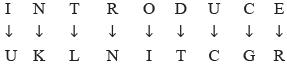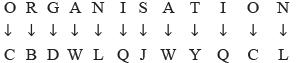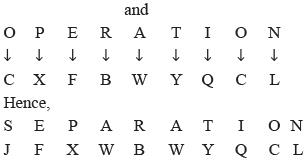Courses

Olympiad Test: Coding Decoding - 2

20 Questions MCQ Test Mathematics Olympiad Class 7 | Olympiad Test: Coding Decoding - 2

Description
Attempt Olympiad Test: Coding Decoding - 2 | 20 questions in 40 minutes | Mock test for Class 7 preparation | Free important questions MCQ to study Mathematics Olympiad Class 7 for Class 7 Exam | Download free PDF with solutions
QUESTION: 1

In a certain code language, BOARD is written as EQBNC. How will the word CLIMB be written in that language?

Solution:

The letters of the word are written in a reverse order and on the group of letters so obtained, the first, third and fifth letters are each moved one step forward while the second and fourth letters are each moved one step backward to get the code.

QUESTION: 2

In a certain code, PLEADING is written as FMHCQMEB. How is SHOULDER written in that code?

Solution:

SHOULDER ⟶ SHOU/LDER ⟶
REDL/SHOU ⟶ ODCK/TIPV.

QUESTION: 3

If in a certain language SHIFT is coded as RFFBO, which word would be coded as LKUMB?

Solution:

The first, second, third, fourth and fifth letters in the word are respectively one, two, three, four and five steps ahead of the corresponding letter of the code.

QUESTION: 4

In a certain code, JANUARY, is written as RYUAANJ. Which word will be written as ERMBCEDE in that code?

Solution:

The word is divided into groups of two letters and first word is divided into one group.

QUESTION: 5

In a certain code, REFRIGERATOR is coded as ROTAREGIRFER. Which word would be coded as NOITINUMMA?

Solution:

The order of letters of the word is reversed in the code. So, reverse the order of the letters in the code to get the word.

QUESTION: 6

In a certain code, O is written as D, A as F, M as I, S as O, N as P, E as M, I as C, P as Q and S as R, then how will COMPANIES be written in that code?

Solution:

Substituting the letters of the given word with their respective codes.QUESTION: 7

If MINERAL is written as QRSTUVW and sound is written as ABCSD, then how will READER be written in the same code?

Solution:

Letter M I N E R A L S O U D
Code Q R S T U V W A B C D
The code for READER is UTVDTU.

QUESTION: 8

If TEACHER is coded as LMKJNMP, then how will HEART be coded?

Solution:

Letter T E A C H R
Code L M K J N P
The code for HEART is NMKPL.

QUESTION: 9

If R is denoted by N, D is denoted by T, I by U, O by I, E by R, T by L, U by C, N by K and C by G, then how will the word INTRODUCE be written?

Solution:

Substituting the letters of the given word with their respective codes,QUESTION: 10

If the word PORTER can be coded as MBNZQN. How can REPORT be written?

Solution:

Letter P O R T E
Code M B N Z Q
The code for REPORT is NQMBNZ.

QUESTION: 11

If the word RASCALS can be coded as BNMZQTI. How can SALSA be written?

Solution:

Letter R A S C L
Code B N M Z T
The code for SALSA is MNTMN.

QUESTION: 12

If in a code language, ORGANISATION is written as CBDWLQJWYQCL and OPERATION is written as CXFBWYQCL, then how is SEPARATION coded?

Solution:QUESTION: 13

In a code language, FACTORIAL is written as DODNGLCFH and DANCE is written as YCJMZ, how can EDUCATION be written in that code?

Solution:

Letter F A C T O R I L D N E
Code D O D N G L C H Y J Z
The code for EDUCATION is ZJDONCGJ.

QUESTION: 14

In a coding system,  SHEEP is written as GAXXR and BLEAT as HPXTN. How can SLATE be written in this coding system?

Solution:

Letter SH E P B L A T
Code GA X R H P T N
The code for SLATE is GPTNX.

QUESTION: 15

In a code language, STARK is written as LBFMG and MOBILE is written as TNRSPJ. How is BLAME written in that code?

Solution:

Letter S T A R K M O B I L E
Code L B F M G T N R S P J
The code for BLAME is RPFTJ.

QUESTION: 16

If MACHINE is coded as 19 – 7 – 9 – 14 – 15 – 20 – 11. How will you code DANGER?

Solution:
QUESTION: 17

If A = 26, SUN = 27, then CAT = ?

Solution:

SUN = S + U + N = 8 + 6 + 13 = 27
CAT = C + A + T = 24 + 26 + 7 = 57

QUESTION: 18

If AT = 20, BAT = 40, then CAT will be equal to.

Solution:

A = 1, B = 2, …… T = 20, …… , Z = 26.
AT = A×T = 1 × 20 = 20; BAT = B × A × T
= 2 × 1 × 20 = 40
CAT = C × A × T = 3 × 1 × 20 = 60

QUESTION: 19

If DEER = 12215 and HIGH = 5645, how will you code HEEL?

Solution:
QUESTION: 20

If O = 16, FOR = 42, then what is FRONT equal to?

Solution:

A = 2, B = 3, ……, Z = 27.
FOR = F + O + R = 7 + 16 + 19 = 42
FRONT
= F + R + O + N + T
= 7 + 19 + 16 + 15 + 21 = 78Use Code STAYHOME200 and get INR 200 additional OFF Use Coupon Code

Track your progress, build streaks, highlight & save important lessons and more!

Similar ContentRelated tests